# How to use the ACOS Function in Excel

COS function is a trigonometric function to calculate the Cosine of the angle of a right angled triangle. Cosine value of an angle of the triangle returns the ratio of the sides i.e. adjacent site ( = x ) / Hypotenuse ( = z ).Cosine of an angle is the angle made by the side and hypotenuse
Cosine (Angle()) = adjacent side ( = x ) / hypotenuse ( = z )
COS () = x / z

So, it means Cosine function returns the ratio of the sides when the angle is given as input. Now if we wish to return the angle when ratio of the sides is given as input, we’ll use the inverse of the Cosine function which is the ACOS function. The above explained representation is shown in below equations

= COS -1( x / z ) { in mathematics }
= ACOS ( x / z ) { in Excel }

ACOS function in excel returns the angle value in radians, the function takes input the ratio of the two sides.
Syntax:
= ACOS ( number )
number : basically the ratio of the two sides in radians. number of the input argument must be between - 1 to + 1.

Let’s understand more about the ACOS function using it in an example: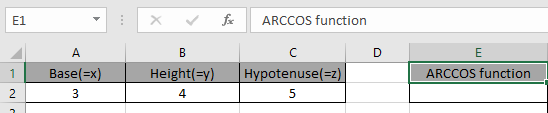Here we have the lengths of sides of the right - angle Triangle having sides as base, height and hypotenuse.

So we need to find the inverse Cosine of the ratio of the sides.

Use the formula:

 =ASIN(A2/C2)

A2/C2 : it returns the ratio of the sides where value of the sides is given in as cell reference.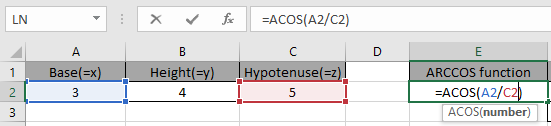Given the ratio of the sides as input to the ACOS function in excel and Press Enter.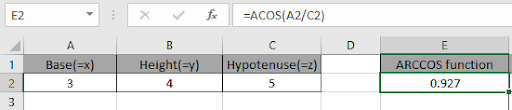As you can see, the ACOS function returns a value in radians.

The above method returns the value in radians. So to convert the value from radians to degrees use the DEGREES function or multiply the result with 180/PI().

Use the formula:

 = DEGREES ( ACOS ( E2 )

OR

 = COS ( E2 ) * 180 / PI()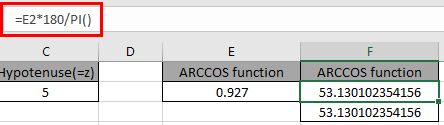The above snapshot gets the result as 53.13 degrees.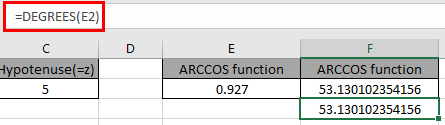The above snapshot use the DEGREES function which takes the input in radians and returns the value in degrees.

Here are some of the observations gathered using the ACOS function.

Note:

1. ACOS function returns in the range of 0.. ( 0 degrees ) to +3.1415.. ( 180 degrees ).
2. The input argument to the function must be between -1 to +1 or else the function returns the #NUM! Error.
3. The function returns #VALUE! Error if the argument to the function is non numeric.

As you can see, we can get the inverse of the Cosine function using ACOS function.

Hope you understood How to use ACOS function in Excel. Explore more articles on Mathematical function like ASIN function and ATAN function here. Please state your query in the comment box below.

Terms and Conditions of use

The applications/code on this site are distributed as is and without warranties or liability. In no event shall the owner of the copyrights, or the authors of the applications/code be liable for any loss of profit, any problems or any damage resulting from the use or evaluation of the applications/code.